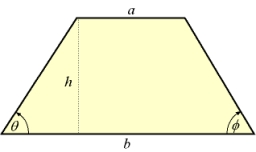# The bases

The bases of the isosceles trapezoid ABCD have lengths of 10 cm and 6 cm. Its arms form an angle α = 50˚ with a longer base. Calculate the circumference and content of the ABCD trapezoid.

o =  22.2229 cm
S =  19.0681 cm2

### Step-by-step explanation:Did you find an error or inaccuracy? Feel free to write us. Thank you!Tips to related online calculators

#### You need to know the following knowledge to solve this word math problem:

We encourage you to watch this tutorial video on this math problem:

## Related math problems and questions:

• The isoscelesThe isosceles trapezoid ABCD has bases of 18 cm and 12 cm. The angle at apex A is 60°. What is the circumference and content of the trapezoid?
• Land - isosceles trapezoidCalculate the building plot's content and perimeter in the form of an isosceles trapezoid with bases 120m, 95m, and height 50m.
• Ratio in trapeziumThe height v and the base a, c in the trapezoid ABCD are in the ratio 1: 6: 3, its content S = 324 square cm. Peak angle B = 35 degrees. Determine the perimeter of the trapezoid
• Isosceles trapezoidThe lengths of the bases of the isosceles trapezoid are in the ratio 5:3, the arms have a length of 5 cm and height = 4.8 cm. Calculate the circumference and area of a trapezoid.
• TrapezoidsIn the isosceles trapezoid ABCD we know: AB||CD, |CD| = c = 8 cm, height h = 7 cm, |∠CAB| = 35°. Find the area of the trapezoid.
• Isosceles triangleCalculate the area and perimeter of an isosceles triangle ABC with base AB if a = 6 cm, c = 7 cm.
• ISO trapezoid v2bases of Isosceles trapezoid measured 20 cm and 4 cm and its perimeter is 55 cm. What is the are of a trapezoid?
• Isosceles trapezoidFind the height in an isosceles trapezoid if the area is 520 cm2 and the base a = 25 cm and c = 14 cm. Calculate the interior angles of the trapezoid.
• IsoscelesIsosceles trapezium ABCD ABC = 12 angle ABC = 40 ° b=6. Calculate the circumference and area.
• DiagonalThe rectangular ABCD trapeze, whose AD arm is perpendicular to the AB and CD bases, has an area of 15 cm square. Bases have lengths AB = 6cm, CD = 4cm. Calculate the length of the AC diagonal.
• Right triangleIt is given a right triangle angle alpha of 90 degrees beta angle of 55 degrees c = 10 cm use Pythagorean theorem to calculate sides a and b
• Isosceles trapeziumCalculate the area of an isosceles trapezium ABCD if a = 10cm, b = 5cm, c = 4cm.
• Right triangleCalculate the missing side b and interior angles, perimeter, and area of ​​a right triangle if a=10 cm and hypotenuse c = 16 cm.
• Isosceles trapezoidCalculate the content of an isosceles trapezoid whose bases are at ratio 5:3, the arm is 6cm long and it is 4cm high.
• Arm and baseThe isosceles triangle has a circumference of 46 cm. Calculate its area if the arm is 5 cm longer than the base.
• Isosceles triangleCalculate the area of an isosceles triangle, the base of which measures 16 cm and the arms 10 cm.
• Isosceles trapezoidFind the area of an isosceles trapezoid, if the bases are 12 cm and 20 cm, the length of the arm is 16 cm